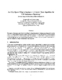## An O(n)-Space O(log n/log log n + f)-Query Time Algorithm for 3-D Dominance Reporting##### Files
CS-TR-4508.ps(179.15 KB)
We present a linear-space algorithm for handling the {\em three-dimensional dominance reporting problem}: given a set $S$ of $n$ three-dimensional points, design a data structure for $S$ so that the points in $S$ which dominate a given query point can be reported quickly. Under the variation of the RAM model introduced by Fredman and Willard~\cite{Fredman94}, our algorithm achieves $O(\log n/\log\log n+f)$ query time, where $f$ is the number of points reported. Extensions to higher dimensions are also reported. (UMIACS-TR-2003-77)